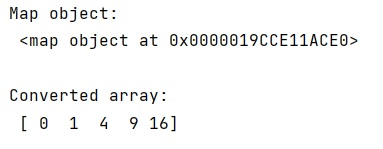# How to convert map object to NumPy array?

Given map object, we have to convert it into a NumPy array in Python.
Submitted by Pranit Sharma, on February 19, 2023

NumPy is an abbreviated form of Numerical Python. It is used for different types of scientific operations in python. Numpy is a vast library in python which is used for almost every kind of scientific or mathematical operation. It is itself an array which is a collection of various methods and functions for processing the arrays.

## Converting map object to NumPy array

Suppose that we are performing some operation using the lambda function and then mapping the result and storing it into some variable. This result is the map object which we need to convert into a numpy array.

For this purpose, we can use numpy.fromiter() where we can pass the map object. It is used to create a new 1-dimensional array from an iterable object. We can also define the data type of the elements of the numpy array which is going to be created.

Let us understand with the help of an example,

## Python code to convert map object to NumPy array

```# Import numpy
import numpy as np

# performing some operation
f = lambda x: x**2

# Creating a map object
seq = map(f, range(5))

# Display map object
print("Map object:\n",seq,"\n")

# Converting map object into numpy array
arr = np.fromiter(seq,dtype='int64')

# Display numpy array
print("Converted array:\n",arr)
```

Output: